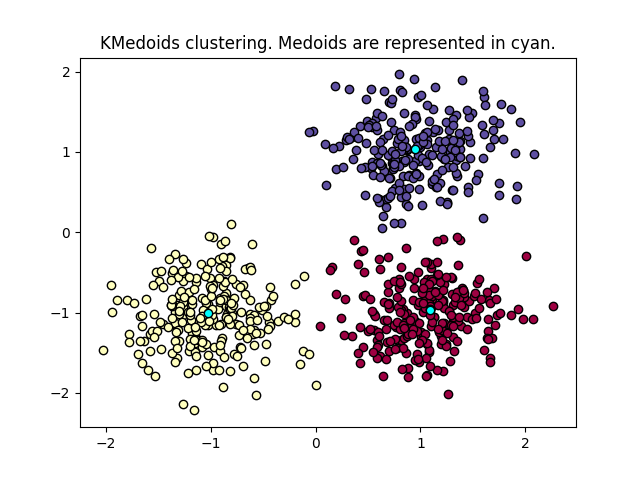# KMedoids Demo¶

KMedoids clustering of data points. The goal is to find medoids than minimize the sum of absolute distance to the closest medoid. A medoid is a point of the dataset. Read more in the User Guide.

```import matplotlib.pyplot as plt
import numpy as np

from sklearn_extra.cluster import KMedoids
from sklearn.datasets import make_blobs

print(__doc__)

# #############################################################################
# Generate sample data
centers = [[1, 1], [-1, -1], [1, -1]]
X, labels_true = make_blobs(
n_samples=750, centers=centers, cluster_std=0.4, random_state=0
)

# #############################################################################
# Compute Kmedoids clustering
cobj = KMedoids(n_clusters=3).fit(X)
labels = cobj.labels_
```

Plot results

```unique_labels = set(labels)
colors = [
plt.cm.Spectral(each) for each in np.linspace(0, 1, len(unique_labels))
]
for k, col in zip(unique_labels, colors):

plt.plot(
xy[:, 0],
xy[:, 1],
"o",
markerfacecolor=tuple(col),
markeredgecolor="k",
markersize=6,
)

plt.plot(
cobj.cluster_centers_[:, 0],
cobj.cluster_centers_[:, 1],
"o",
markerfacecolor="cyan",
markeredgecolor="k",
markersize=6,
)

plt.title("KMedoids clustering. Medoids are represented in cyan.")
```Out:

```Text(0.5, 1.0, 'KMedoids clustering. Medoids are represented in cyan.')
```

Total running time of the script: ( 0 minutes 0.203 seconds)

Gallery generated by Sphinx-Gallery